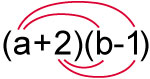Mathematics for science and technology

Start this free course now. Just create an account and sign in. Enrol and complete the course for a free statement of participation or digital badge if available.

Free course

# 2.3 Brackets in mathematics

As you have seen, brackets are used to indicate, and clarify, the order in which a numerical calculation should be carried out. This is also true when you are using symbols, as in algebra.

So, for example considering 2(2n + 5) you should first multiple out the brackets. This means that all of the expression inside the brackets is multiplied by the figure outside them – a crucial idea to remember.

Thus

(Note, as a convention, 2(2n + 5) is preferable to (2n + 5)2, although, since it doesn’t matter what order you multiply two numbers in, they are equivalent.)

Here’s another example:

The important point to note in this example is that everything in the bracket was multiplied by –2, not just 2.

Things become more complicated when you need to multiply two expressions both within brackets. For this you have to multiply each term, in both brackets by each other.

Let’s consider this example:

(a + 2)(b – 1)

It is easier to see the individual steps required by drawing lines between the terms in the expressions.If a = b

Then the expression becomes:

a2 + a – 2

Now have a go yourself in this activity.

## Activity 2 Removing brackets

Expand each of the following expressions by removing the brackets. Then (if possible) collect like terms.

1. 2(2x – y)
2. –2(a + 2b – c)
3. 4(r + 6s) – (4s – r)
4. x(2x – 3) – 2x(5 – 2x)
5. (x – 2)(x + 3)
6. (r + t)2
7. (a – 4)(a + 4)

1.       2(2x – y) = 4x – 2y
2.       –2(a + 2b – c) = –2a – 4b + 2c
3.
4.
5.
6.
7.
8.

You should now feel more confident with simplifying algebraic expressions – don’t worry if some of these felt quite complex. The more practice you get the easier this will get.

Now it is time to look at how to rearrange equations.

MST_1